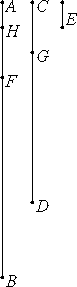# Proposition 1

When two unequal numbers are set out, and the less is continually subtracted in turn from the greater, if the number which is left never measures the one before it until a unit is left, then the original numbers are relatively prime.

The less of two unequal numbers AB and CD being continually subtracted from the greater, let the number which is left never measure the one before it until a unit is left.VII.Def.12

I say that AB and CD are relatively prime, that is, that a unit alone measures AB and CD.

If AB and CD are not relatively prime, then some number E measures them. Let CD, measuring BF, leave FA less than itself, let AF, measuring DG, leave GC less than itself, and let GC, measuring FH, leave a unit HA.

Since, then, E measures CD, and CD measures BF, therefore E also measures BF.

But it also measures the whole BA, therefore it measures the remainder AF. But AF measures DG, therefore E also measures DG. But it also measures the whole DC, therefore it also measures the remainder CG.

But CG measures FH, therefore E also measures FH. But it also measures the whole FA, therefore it measures the remainder, the unit AH, though it is a number, which is impossible.

VII.Def.12

Therefore no number measures the numbers AB and CD. Therefore AB and CD are relatively prime.

Therefore, when two unequal numbers are set out, and the less is continually subtracted in turn from the greater, if the number which is left never measures the one before it until a unit is left, then the original numbers are relatively prime.

Q.E.D.

## Guide

#### Example

Following the algorithm described in this proposition, the numbers 54 and 85 are relatively prime as the following computations show.

 85 – 2 · 31 = 23 31 – 23 = 8 23 – 2 · 8 = 7 8 – 7 = 1

#### Antenaresis, the Euclidean algorithm

Modern terminology uses the word “divides” rather than “measures,” and the notation a | b is used to abbreviate the phrase "a divides b."

This proposition assumes that 1 is the result of an antenaresis process. Antenaresis, also called the Euclidean algorithm, is a kind of reciprocal subtraction. Beginning with two numbers, the smaller, whichever it is, is repeatedly subtracted from the larger.

If the initial two numbers are a1 (AB in the proof) and a2 (CD), with a1 greater than a2, then the first stage is to repeatedly subtract a2 from a1 until a remainder a3 (AF) less than a2 is found. That can be stated algebraically as

a1 – m1 a2 = a3

where m1 is the number of times that a2 was subtracted from a1.

The next stage repeatedly subtracts a3 from a2 leaving a remainder a4 (CG):

a2 – m2 a3 = a4.

For the hypotheses of this proposition, the algorithm stops when a remainder of 1 occurs:

an-1 – mn-1 an = 1.

(In Euclid’s proof, an is a5 which is AH.) The conclusion is that a1 and a2 are relatively prime.

The proof is not difficult. It depends on the observation that if b divides (that is, measures) both c and d, then b divides their difference c – d. So, if some number b divides both a1 and a2, then it divides the remainder a3, too. And since it divides both a2 and a3, it divides the remainder a4. And so forth, with the final conclusion that b divides the last remainder 1.

Since there is no number b (and by “number” is meant a number greater than 1) which divides 1, there is no number that divides both a1 and a2. Therefore a1 and a2 are relatively prime.

Compare this proposition to X.2, a somewhat analogous statement about magnitudes.

This proposition is used in the proof of the next one.